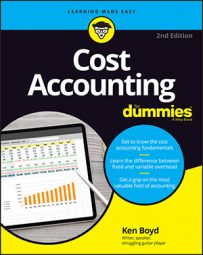##### Cost Accounting For DummiesWhen cost accounting, you use the weighted average costing method to calculate costs in a process-costing environment. Now incorporate weighted average analysis into calculating spoilage costs.

To get super-psyched for the weighted average method, keep these points in mind:

• To keep it simple, you analyze only the material units and material costs for a product.

• Assume that material costs go into production at the beginning of the period. Material is 100 percent complete in any ending work in process.

• The weighted average method calculates units and costs on work done to date. The cost includes work performed in the preceding period (beginning WIP) and in the current period.

Here are the steps you take to implement process costing:

1. Account for the physical units you produce.

2. Compute equivalent units, based on percentage of completion.

3. Total your costs and then calculate cost per equivalent unit.

4. Multiply your equivalent units by cost per equivalent unit.

You’re going through the same process. You’re simply adding in the impact of spoilage.

## Cost accounting for physical units

Say you manufacture an electric kitchen wall oven. Your total production cost is \$1,500 per oven. A portion of that cost is for materials (plastic, steel, and aluminum). You use the weighted average method to account for costs, including the cost of spoilage. As you start the analysis for May, consider these factors:

• You include normal spoilage units as completed units. That means that normal spoilage units will be part of the equivalent unit calculation.

• Normal spoilage is expected to be 5 percent of production. That percentage is based on past production results and your product knowledge.

• The cost of abnormal spoilage won’t be attached to the product. Instead, those costs are posted to a “loss for abnormal spoilage” account. Abnormal spoilage isn’t part of the cost of goods manufactured.

Start by accounting for the physical units. Check out the table.

Weighted Average — Physical Flow of Units (May)
Material Cost Analysis Units Equivalent Units
Work in process, beginning inventory (5/1) 100
Units started during May 600
Units to account for 700
Completed and transferred out during May 400 400
Normal spoilage (5 percent of good units) 20 20
Abnormal spoilage (2.5 percent of good units) 10 10
Work in process, ending inventory (5/31) 270 270
Units accounted for 700 700

For these high-end ovens, material is introduced at the beginning of production. For your analysis, assume that material costs are 100 percent complete. As a result, the equivalent units are equal to the physical units. The 700 units for which you need to account equal the 700 units accounted for.

## Cost accounting for equivalent units

Here are the material costs for making ovens for the period:

Total costs = cost of beginning inventory + costs added during the period
Total costs = \$80,000 + \$400,000
Total costs = \$480,000

To calculate equivalent units, divide total costs for the period by the total equivalent units. Here’s the calculation:

\$480,000 total costs ÷ 700 equivalent units = \$685.71 cost per equivalent unit

This table assigns the \$480,000 total cost to units produced. The table multiplies equivalent units by the cost per equivalent unit (\$685.71).

Weighted Average — Equivalent Units (May)
Equivalent Units Cost Per Unit Total Cost
Completed, transferred 400 \$685.71 \$274,286
Normal spoilage 20 \$685.71 \$13,714
Cost of good units 420 \$685.71 \$288,000
Abnormal spoilage 10 \$685.71 \$6,857
Work in process, ending 270 \$685.71 \$185,143
Totals 700 \$480,000

Note in this table that the cost of good units includes both units transferred out and the normal spoilage. The total units (700) agree with the total in the first table. The total costs assigned (\$480,000) agree with the total cost calculation in this table.

Normal spoilage cost (\$13,714) is attached to cost of goods manufactured. Abnormal spoilage cost (\$6,857) is recognized as a loss — not attached to the cost of goods.

You’ve assigned total material costs to the units you worked. To complete costs for the full product, you perform the same analysis on conversion costs. You finish up by adding material costs to conversion costs. That total is your full product cost for the ovens.